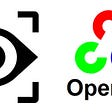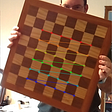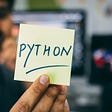# Day two in Machine Learning: k-nearest neighbours.

The k-nearest neighbours’ algorithm (kNN) is a non-parametric machine learning method used for classification and regression. It is non-parametric because the model does not learn any parameters to make correct predictions. Instead, it will look at closest training examples (the number of examples depends on the k selected by the user) in feature space. When used for classification, the output is a class group. An object is classified by a plurality vote of its neighbours, with the item being assigned to the class most common among its k nearest neighbours. When used for regression, the output is the average of the values of k nearest neighbours. This article shows how it works and how it can be developed in python. Link to the source code is available at the end and this is the link to the previous post.

The graph above (Fig. 1) shows 28 samples of data representing 28 people and their colour preferences. A red dot is a person who likes the colour red, green dot green colour and blue dot blue colour. For each person, we know the annual salary and the age so that we can draw the above scatter plot. The goal is to predict what is the favourite colour of a person that is 55 years old and with an annual income of 80k. If we think for a moment on how we would get a solution I assume that we will look at the closest points in the graph, count the majority of colours near the new data point and assign that colour to it. In this case, it will be red. KNN works in the same way for classification tasks and would do the same but calculating the average value of the neighbours in regression tasks.

Consider the simple example in figure 2. There are 2 green squares and 3 red triangles in our dataset. We want to predict the class of the new data point in grey. First, KNN will calculate the distances of the new data point to all the other data points and store in an internal dictionary (the distances are 3, 2.8, 0.5, 2.4 and 5.5). After the distance calculation, KNN will take the dictionary and sort it with the shorter distances first and the respective item associated (0.5: square, 2.4: triangles, 2.8: triangles, 3: square, 5.5: triangles). In this example, the user has decided to implement the KNN with k equal to 3. This means that KNN will look at the first 3 items of the sorted dictionary and count the items per classes. In the first 3 items of the sorted dictionary, there are 1 square and 2 triangles so KNN will assign the class triangle to the new data point because the triangle is the majority class in the 3 selected samples.

The question could be: what is the right number of K to use? By looking at the example there are a total of 6 squares and 5 triangles. The probability that a new point can be a square is a bit higher and if we choose a very large number of K that will consider all the training data the output of KNN will be always the majority of classes considering the entire dataset. If we take a low number of K the prediction will be sensitive to outliers. If we choose 5 the prediction will be square but if we choose 3 the prediction will be a triangle. Assuming the two red triangles in the centre are outliers choosing a k equal to 3 might not be the best choice. In practice, the best way to select the correct value of K is to try different values and select the one that produces the most consistent accuracy compared with different testing. Notice that the comparison is between testings and not training and testing since in KNN (as a non-parametric algorithm) there is not a real training phase. Training and prediction with KNN happen at the same time when KNN perform all the steps mentioned earlier.

Figure 4 shows the scatter plot of the dataset used for the following example where we build a KNN from scratch following the steps of the algorithms in details. Full code here.

Above there is the function that can be used to calculate the distances of points in the features space. The length is the number of dimension of the dataset and it will include the target variable. So below we subtract the length by 1. Euclidian distance is one option but there are several other alternatives: Scikit Learn provides a parameter called p to change the distance metrics depending on tasks.

The “getNeighbours” is the function that will use the distances to create the sorted dictionary with the k number of points sorted based on their distances to the new data point that we want to predict.

The function getResponse will look into the dictionary, calculate the majority of votes and output the predicted value. The first item key of the sorted response.

In order to calculate the accuracy of the algorithm when we test it with several new data points, we can use the above function. It will simply store how many correct and how many wrong predictions the algorithm did, compared to a true label that we kept for testing purpose.

The main programme will run the entire algorithm. I find learning an algorithm by code sometimes easier than by theory, but there are plenty of libraries in different programming languages that implement the KNN for us:

## More from Analytics Vidhya

Analytics Vidhya is a community of Analytics and Data Science professionals. We are building the next-gen data science ecosystem https://www.analyticsvidhya.com

## Weighted Envy-Freeness in Indivisible Item Allocation## Training Generative Adversarial Network (codes provided)## Review: DIS — Dual Image Segmentation (Semantic Segmentation)## RL—Markov Decision Processes## OpenCV: Taste of feature extraction in Computer Vision.## AI in Medicine — Majority decision isn’t always right## Review — Deep Captioning with Multimodal Recurrent Neural Networks (m-RNN)## Autonomous Car Part 5Data Scientist

## Python Fundamental for Data Science## How I Did and How You Can Get Started On ML## Welcome to my first Data Science Project write-up!